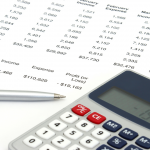# Calculator for CPA examWhat calculator can I take into the CPA exam?

You can take any calculator in to exam, including a financial calculator, as long as it does not have any data storage or recording functions.

CPA Australia will not provide you with a list of appropriate models, because there are so many to choose from. So, there is little point in contacting them or posting a question in MYOL (My Online Learning) student discussion forum.

## Is my calculator for my CPA exam is appropriate?

There is a simple test: Can I do all the calculations that I have found throughout the study guide?

If the answer is yes – then, the calculator will be adequate.

You can buy a reasonable calculator for under \$30.

Here is an example of different types:

http://www.officeworks.com.au/shop/officeworks/c/office-supplies/calculators

March 12, 2016

### 3 responses on "Calculator for CPA exam"

1.Hi Courtney,

I have the similar one with the one you suggested but it doesnot have the power functions? how can we calculate the return generated from paying invoices early?

Regards,

Quyen

2.Hi Quyen,

Thanks for your message. For SMA, I’d strongly recommend using a calculator with the power function. This will be helpful for the discount saving formula, as well as the EOQ formula and then NPV calculations in Module 5.

To calculate the discount saving formula without the using the power functions of a calculator would most likely require estimation. Without going into detail on the long mathematical formulas, you would be able to approximate the answer on page 90 by calculating: (1.0526 x 1.0526 x 1.0526 x 1.0526 x 1.0526 x 1.0526 x 1.0526 x 1.0526 x 1.0526 x 1.0526 x 1.0526 x 1.0526 x 1.00877) – 1. Here, the first 12 multiplications effectively take 1.0526 to the power of 12. Then, the last figure of 1.00877 represents (1 + (0.1667 x 0.0526). This calculation totals 86.62%, which approximates the 86.58% result on page 90.

Cheers,
Russell

3.Hi

I normally use the HP10Bii financial calculator but noticed that there is also a HP10Bii+ available. Is the HP10Bii+ allowed to be used in exams?

Best regards